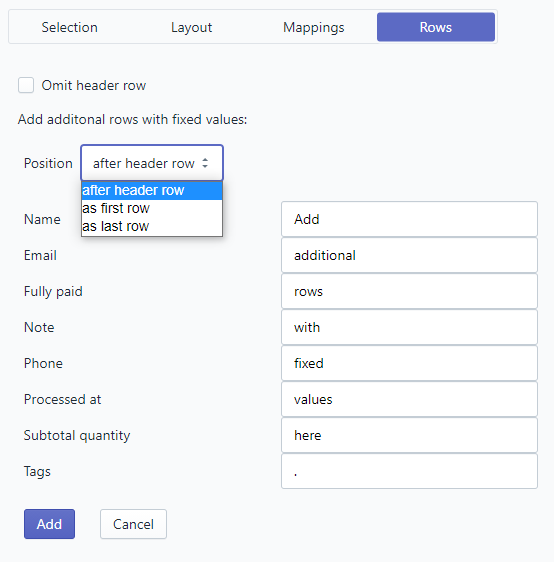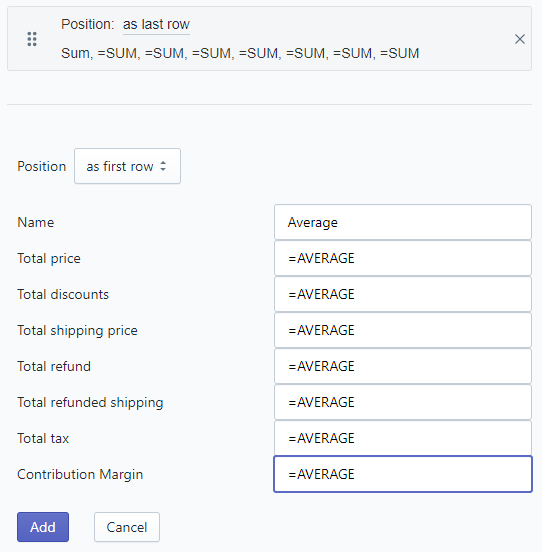# Rows

The "Row" tab allows you to customize:

• "Omit header row": No header row will be in the ouptut file (Excel, CSV)

• as first row
• as last row

Screenshot:## Use Excel formulars to calculate sum, average, ...

This will work only with file format Excel. And it will work only for rows that are "as last row".

You can use any Excel formular that will calculate the content from the column above (like SUM or AVERAGE do). And as in Excel start your input with a "=" like: "=SUM", "=AVERAGE" or "=COUNT"

You can add as many "as last rows" as you want - like in this example: